# Solution assignment 15 Quadratic equations (factorizing)

### Assignment 15

Try to solve by factorizing: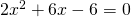### Solution

In this equation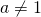, but both sides can be divided by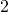, yielding the equation: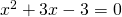Next we have to find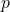and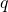such that: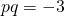and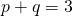There are no whole numbersandthat satisfy these equations. This does not mean that the equation has no solutions. In this case the equation has two solutions (because the discriminant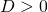), and we apply the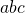-formula, see Quadratic equations (abc-formula).

0